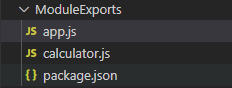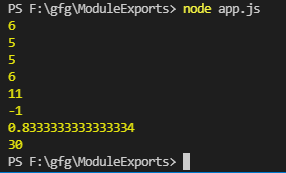# How to write code using module.exports in Node.js ?

• Difficulty Level : Expert
• Last Updated : 23 Nov, 2021

module is a discrete program, contained in a single file in Node.js. They are tied to files with one module per file. module.exports is an object that the current module returns when it is “required” in another program or module.

We are going to see a simple code like the calculator to learn how to use module.exports in Node.js. I will walk you through step by step.

Step 1: Create two files “app.js” and “calculator.js” in your project folder. And initialize the project through npm in the terminal.

npm initAfter step 1

Step 2: Now we have a nodeJS based project with “app.js” as its entry point. Now let’s first code “calculator.js“. We will create a class named Calculator in it. It would have several member functions namely:

• preIncrement: For the prefix increment operation
• postIncrement: For the postfix increment operation
• preDecrement: For the prefix decrement operation
• postDecrement: For the postfix decrement operation
• subtract: For subtraction of two numbers
• multiply: For multiplication of two numbers
• division: For division of two numbers

And at last, we export the Calculator Class through the module.exports

## calculator.js

 `// ***** calculator.js file *****``class Calculator {``     ` `    ``// Constructor to create object of the class``    ``Calculator() {``         ` `    ``}`` ` `    ``// Prefix increment operation``    ``preIncrement(a) {``        ``return` `++a;``    ``}`` ` `    ``// Postfix increment operation``    ``postIncrement(a) {``        ``return` `a++;``    ``}`` ` `    ``// Prefix decrement operation``    ``preDecrement(a) {``        ``return` `--a;``    ``}`` ` `    ``// Postfix decrement operation``    ``postDecrement(a) {``        ``return` `a--;``    ``}`` ` `    ``// Addition of two numbers``    ``add(a, b) {``        ``return` `a + b;``    ``}`` ` `    ``// Subtraction of two numbers``    ``subtract(a, b) {``        ``return` `a - b;``    ``}`` ` `    ``// Division of two numbers``    ``divide(a, b) {``        ``return` `a / b;``    ``}`` ` `    ``// Multiplication of two numbers``    ``multiply(a, b){``        ``return` `a * b;``    ``}``}`` ` `// Exporting Calculator as attaching``// it to the module object``module.exports= Calculator;`

Step 3: Now let’s code the “app.js” file.

First, we will import the Calculator class by requiring it from the “calculator.js” file. Then we create an object  “calc” of Calculator class to use its member methods.

## app.js

 `// ***** app.js file  ******``// Importing Calculator class``const Calculator = require(``'./calculator.js'``);`` ` `// Creating object of calculator class``const calc = ``new` `Calculator();`` ` `/* Using all the member methods ``defined in Calculator */`` ` `console.log(calc.preIncrement(5))`` ` `console.log(calc.postIncrement(5))`` ` `console.log(calc.preDecrement(6))`` ` `console.log(calc.postDecrement(6))`` ` `console.log(calc.add(5, 6))`` ` `console.log(calc.subtract(5, 6))`` ` `console.log(calc.divide(5, 6))`` ` `console.log(calc.multiply(5, 6))`

Step to run the application: Open the terminal and type the following command.

`node app.js`Output

In this way, you can use module.exports in node.js. In place of class here you can pass any variable, literal, function, or object. And later import it by using require()

My Personal Notes arrow_drop_up